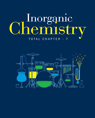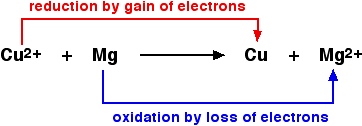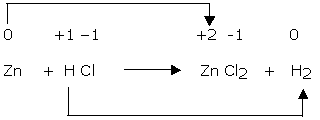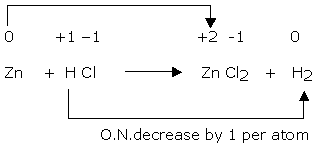×#### Thank you for registering.

One of our academic counsellors will contact you within 1 working day.

Click to Chat

1800-1023-196

+91-120-4616500

CART 0

• 0

MY CART (5)

Use Coupon: CART20 and get 20% off on all online Study Material

ITEM
DETAILS
MRP
DISCOUNT
FINAL PRICE
Total Price: Rs.

There are no items in this cart.
Continue Shopping```Oxidation Reduction

Table of Content

Oxidation and Reduction in Terms of Oxygen Transfer

Oxidation and Reduction in Terms of Hydrogen Transfer

Oxidation and Reduction in Terms of Electron Transfer

Oxidizing and Reducing Agents​

Balancing Oxidation-Reduction Reactions

Oxidation Number Method

Oxidation and Reduction in terms of oxygen transfer

Oxidation is defined as the gain of oxygen

Reduction is defined as loss of oxygenFig. 1. Oxidation and reduction in terms of oxygen transfer

When oxidation and reduction occurs together, this is known as Redox Reaction.

Oxidation and Reduction in terms of Hydrogen Transfer

Oxidation involves the loss of hydrogen.

Reduction involves gain of hydrogen.

Oxidation and Reduction in Terms of Electron Transfer

Oxidation is defined as loss of electrons.

Reduction is defined as gain of electrons.

The easiest method to learn oxidation and reduction is LEO-GER/OIL-RIG system.

OILRIG stands for Oxidation Is Loss and Reduction Is Gains. IN ELECTRONS.

LEOGER (leo the lion says grr) stands for Loss of Electrons = Oxidation while Gain in Electrons = Reduction.Fig. 2. Oxidation and reduction in terms of electron transfer

Oxidizing and Reducing Agents

Oxidizing agent is a substance that oxidizes another substance but itself gets reduced. For example, Sulphuric acid, oxygen, ozone etc.

Reducing agent is a substance which reduces another substance but itself gets oxidized. For example, hydrogen, lithium, iron, zinc etc.Fig. 3. Oxidizing and Reducing agents

Balancing Oxidation-Reduction Reactions

The method used to balance the redox reactions is called the Half Equation Method or ion electron method. Each equation is balanced by adjusting coefficients on both the sides, that is, reactant as well as product side.

This can be explained using an example given below-

Cu+(aq) + Fe(s)→Fe3+(aq) + Cu(s)

Separate the reactions into half reactions

Cu+(aq) + e−→Cu(s)

Fe3+ (aq)+3e−→Fe(s)

Balance the electrons in both the equations. In this case multiply the first half equation by 3.

3Cu+(aq) +3e−→ 3Cu(s)

Fe(s) →Fe3+(aq) +3e−

Add both the equations and cancel the number of electrons and balanced equation will be-

3Cu+ (aq)+ Fe(s)→3Cu(s)+ Fe3+ (aq)

Oxidation Number Method

This can be explained step by step wise using an example:

Step 1

The skeleton equation is:

Zn + HCl → ZnCl2 H2

Step 2

Oxidation number of various atoms involved in the reaction:

0 + 1 – 1 + 2 –1 0

Zn + HCl → Zn Cl2 + H2

Step 3

The oxidation number of zinc has increased from 0 to +2 while that of hydrogen has decreased from +1 to 0. However, the oxidation number of chlorine remains same on both sides of the equation. Therefore, zinc is reducing agent while HCl is oxidizing agent in reaction:Step 4

The increase and decrease in oxidation number per atom can be indicated as: O.N. increases by 2 per atomStep 5

The increase in oxidation number of 2 per atom can be balanced with decrease in oxidation number of 1 per atom if Zn atoms are multiplied by 1 and HCl by 2. The equation will be:

Zn + 2HCl → ZnCl2 + H2

Watch this Video for more reference

Oxidation Reduction
```### Course Features

• 731 Video Lectures
• Revision Notes
• Previous Year Papers
• Mind Map
• Study Planner
• NCERT Solutions
• Discussion Forum
• Test paper with Video Solution